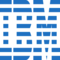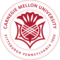A Simple Explanation of the Bag of Words (BoW) Model

# A Simple Explanation of the Bag of Words (BoW) Model

clickHere
Updated on Jan 13, 2023 16:13 IST

In this article, we will explore all that there is to know about Bag of Words (BOW) Model.

The Bag of Words (BoW) Model is a Natural Language Processing technique for text modeling. In this blog, we will learn about why we use BoW model the and the concept behind it with explanations. Also, we will learn about its implementation in Python and Skilearn.

## What is Bag of Words (BoW) in NLP?

The Bag of Words model is an NLP technique for text modeling. It is a method of feature extraction that happens with text data. The approach behind this technique is simple and flexible for extracting features from documents.

A BoW Model is a text representation that describes the occurrence of words within a particular document. We should keep track of word counts and disregard the grammatical details and the order of words.

Words collected together are a ‘Bag’ of words, as they will discard any information about the structure or order of words. The model will focus only on the words and the number of occurrences it has on the document.

## Why is the BoW algorithm used?

Why do we need this algorithm for simple text? This thought might arise to us all. One of the main problems with text is that it is unstructured. But machine learning algorithms prefer well-defined, structured, and fixed-length inputs.

Using the BoW technique, we can convert variable-length texts into fixed-length vectors. Also, at a granular level, the machine learning model works with numerical rather than textual data. So, when using the BoW technique, we can convert text to its equivalent number vectors.

You can also explore: Introduction To Backtracking Algorithm

## Where is BoW used?

We often use the BoW model in Information Retrieval and Natural Language Processing. It is used in methods like document classification, where the frequency of occurrence of each word is a feature that is training a classifier.

The BoW model can also be used for computer vision. The most practical application of the BoW model is as a tool for feature generation. After transforming the text into a ‘bag of words’, we can calculate several measures to characterize the text.

You can also explore: Curse of Dimensionality

The most common feature calculated from the BoW model is term frequency. It is essentially the number of occurrences a term has in a document. Term frequency is not majorly the best representation of the text, but it finds successful applications in email filtering areas.

Term frequency is not the best representation as common words are always there in a document, and their frequency will be higher. Though it shows that we have a high raw count, it doesn’t indicate the corresponding word is important.

The popular method for dealing with this problem is normalizing the term frequencies by weighting a term by the inverse of document frequency. Also, for the classification task, we can use supervised algorithms for accounting for the class label of a document. We can even use binary weighting to solve these problems.

You can also explore: KNN Algorithm in Machine Learning

## How does BoW work, and how do we implement it?

Here are the steps involved when we want to implement the Bag of Word Model:

• Preprocess the data: We should convert the text into a lowercase letter, and we should remove all non-word characters and punctuation.
• Finding the frequent words: The vocabulary should be defined by finding the frequency of each word in the document. Each sentence should be tokenized into words, and we should count the number of occurrences of the word.
• Model construction: We should construct the model by building a vector to determine if a word is a frequent word. If it is a frequent word, it can be set as 1, else 0.
• Output: We can now generate the output.

## Understanding the BoW Model with an example

Here is an example to help us understand more about the BoW model:

#### 1. Data Collection:

We should consider some lines of text as different documents that need to be vectorized:

• The cat danced
• Cat danced on chair
• The cat danced with a chair

#### 2. Determine the vocabulary:

The vocabulary is the set of all words found in the document. These are the only words that are found in the documents above like the cat, on, a, the, table, hat, and with.

#### 3. Counting:

The vectorization process will involve counting the number of times every word appears:

This will generate a seven-length vector for each document.

• The cat danced: [1,1,1,0,0,0,0]
• Cat danced on a chair: [1,1,1,1,1,1,0]
• The cat danced with a chair: [1,1,1,1,1,1,1]

Now we can see that the BoW vector only has information about what words occurred and how many times with any contextual information or where they occurred.

#### 4. Managing vocabulary:

With the above example, we can see that as the vocabulary grows, so does the vector representation. When we consider large documents, the vector length can stretch up to millions.

As every document has a few known words, that will create a lot of empty spots with 0s called sparse vectors. When modeling becomes cumbersome with traditional algorithms, there are cleaning methods for reducing the vocabulary size. It will include ignoring the punctuations, fixing the misspelled words, and ignoring the common words like ‘a’, ‘of’, etc.

#### 5. Scoring words:

Scoring words is attaching a numerical value for marking the occurrences of the words. In the above example, scoring was binary: it means the presence or absence of the words. There are other methods as well:

Counts: The number of times each word appears in a document.

Frequencies: Frequencies are the calculation of word frequency in a document in contrast to the total number of words in that document.

You can also explore: Naïve Bayes Algorithm in Machine Learning

## Implementing BoW with Python

Now, let’s go down with the concepts of implementing the BoW model with Python using the below steps:

1. Preprocessing the data:

We should first preprocess the data and tokenize the sentences. We should also transform words into the lower case to avoid word repetition.

The below snippet will help us with the above process:

2. Assigning an index of the words:

Now, we can create an indexed dictionary for assigning a unique index for each word. We can use the below snippet to understand how:

3. Defining the Bag of Words Model’s function:

Finally, we can define the Bag of Word’s function for returning a vector representation of our input sentence using the below code:

4. Testing our model:

With the complete implementation over, we can now test the functionality of our model using the below command:

## What is Tf-Idf?

Term Frequency-Inverse document frequency is a numerical statistic that has the reflecting purpose of how important a word is to a document in a collection of words. TF is a measure of how frequently a word appears in a document.

IDF is a measure of how important that word is to the document. The IDF value is vital as simply computing the TF is not sufficient enough for understanding the importance of the meaning of the words.

## Difference between BoW & Tf-Idf

The major difference between the BoW model and Tf-Idf is that:

The BoW creates a series of vectors that contains the occurrences of a word in the document. Whereas the Tf-Idf holds information on the more important words and the less important ones separately.

Also, interpreting the BoW vector is easy. But, Tf-Idf tends to perform better in machine learning models, so the interpretations might be a bit harder.

## Advantages of the BoW Model

The most significant advantage of the BoW model is it is simple and easy to use. We can use it to create an initial draft model before proceeding with more sophisticated word embeddings.

## Disadvantages of the BoW Model

Here are some disadvantages of the BoW model:

Semantic Meaning:

The basic BoW model doesn’t consider the word’s meaning in the document. It will completely ignore the context in which it is used. We might use the same word in different places based on the context or nearby words.

Vector Size:

For a larger document, the vector size can be huge, which results in a lot of computational time. We should ignore words based on relevance to our use case.

## Wrapping Up

In the blog, we have learned about Bag of Words and its representation in NLP. We are repacking our data into a cluster of tokens using certain paradigms. It will help the model get a basic understanding of the sentence and its meaning.

The BoW approach is limited in its accounting ability for meaning and context. Naturally, to represent sentences as vocabulary occurrences is ineffective for dealing with homonymy and polysemy.

Its inability to account for syntactic dependencies and non-standard text points will not make it a stronger algorithm. But in context with the growth of NLP, this technique has opened various pushes in representational learning to make it a pivotal part of NLP.

Author: Aswini R

## FAQs

What type of technique does BoW provide?

The technique used in BoW Model is the extraction of features from text data. It is a simple technique. The main idea is to represent each sentence as a bag of words, disregarding paradigms and grammar.

Is the BoW Model good?

The Bag of Words (BoW) model is simple to understand and implement. The BoW model successfully rectifies problems like text classification, language modeling, and document classification.

What are the steps of the BoW Model?

Here are the steps for implementing the BoW Model: u25cf Collect data u25cf Design the vocabulary u25cf Create document vectors

Is BoW a feature engineering technique?

Yes, BoW Model is a feature engineering technique. The Bag of Words Model is a feature extraction technique that will convert text data into numerical vectors known as features. Those numbers are the count of every token or word in the document.

How can we use BoW in NLP?

The BoW Model is an orderless documental representation where only the word counts matter. For example, we can take the sentence, u2018Martha likes to watch movies. Martin like movies too.u2019. In this sentence, the BoW representation will not reveal that the verb likes always means the same. This model will help us find the difference and find the exact meaning.

Is BoW a neural network?

Yes, the BoW model is a way to extract features from the text so we can use the text input in machine learning algorithms like neural networks.

What is the main idea behind the BoW model?

The BoW model is a simplifying representation used in NLP and IR. In this model, we will represent a document as a bag of its words, disregarding its grammar and punctuation.

clickHere

This is a collection of insightful articles from domain experts in the fields of Cloud Computing, DevOps, AWS, Data Science, Machine Learning, AI, and Natural Language Processing. The range of topics caters to upski... Read Full Bio

## Trending Data Science CoursesMachine Learning
Coursera4.6Calculus for Machine Learning and Data Science
Coursera4.8## Top Picks & New Arrivals